• 递归法： 1）定义： 递归就是自己调用自己。 2）另外： 能不用递归就不要用递归，因为递归其实就是系统为你压栈了，系统会把所有的东西都压栈（不管你用不用得到），所以造成了递归耗内存。可以用循环来代替递归...
递归法：
1）定义：
递归就是自己调用自己。
2）另外：
能不用递归就不要用递归，因为递归其实就是系统为你压栈了，系统会把所有的东西都压栈（不管你用不用得到），所以造成了递归耗内存。可以用循环来代替递归，自己实现压栈，压入自己需要用到的东西就好。
3）如何计算递归的时间复杂度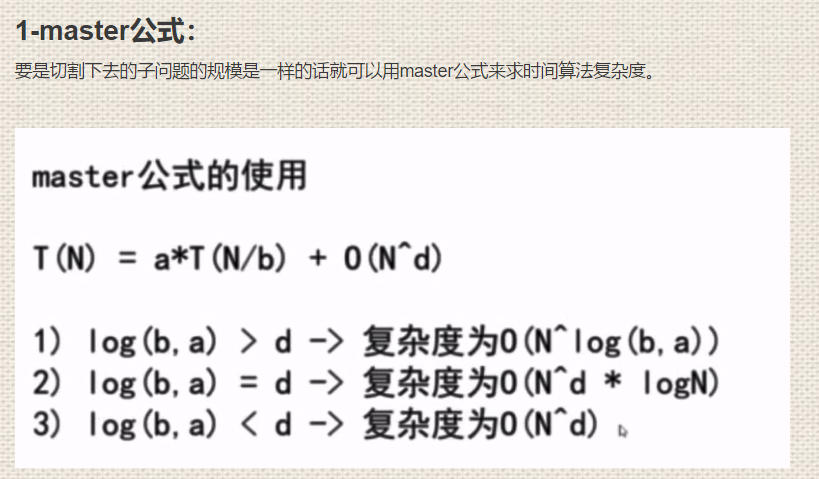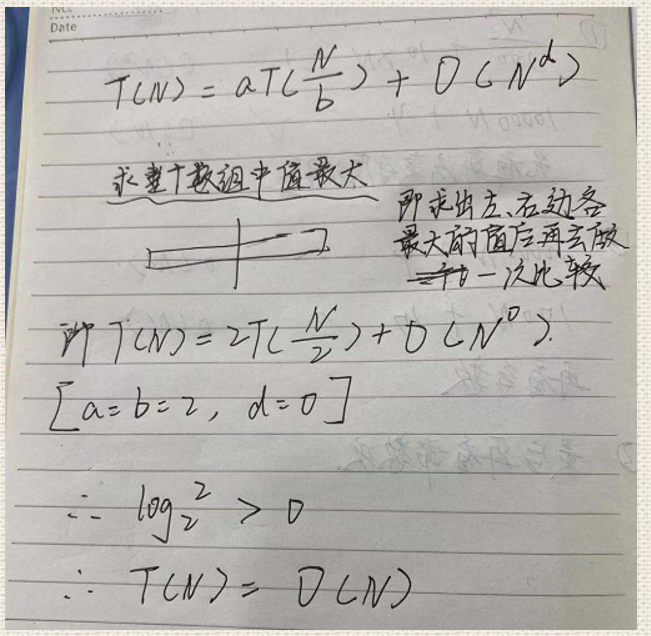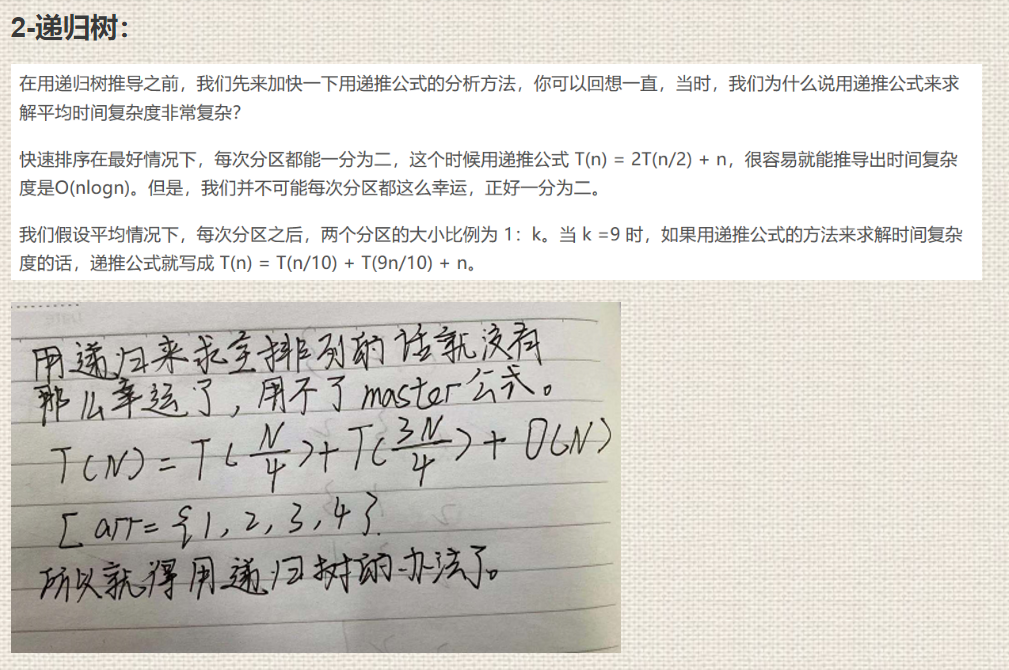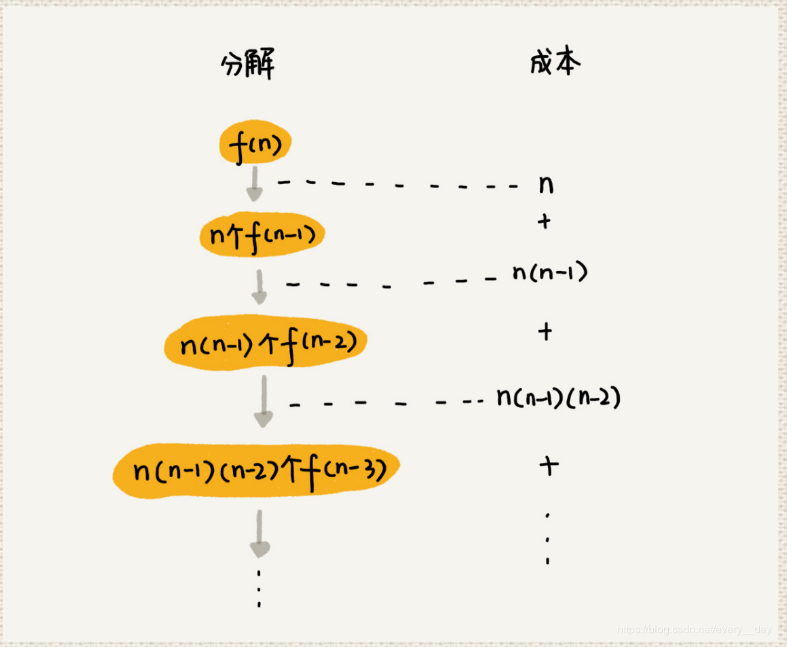4）例子：
（1）问题描述：
求全排列。
（2）基本思路：
就是第一层perm固定了第一个元素arr，第二层固定了第二个元素arr，以此类推，到最后一个的时候就输出数组。
（3）Java代码实现：
public class digui {

public static  void main(String[] args) {
int arr[]= {1,2,3,4};
perm(arr,0,arr.length-1);//求得/打印出 数组arr从0到arr.length-1的全排列

}

public static void swap(int arr[],int x, int y) {
int temp = arr[x];
arr[x]=arr[y];
arr[y]=temp;

}

public static void perm(int arr[],int begin ,int end) {
if(end==begin) {
for(int i =0;i<=end;i++) {
System.out.print(arr[i]+" ");
}
System.out.println();//用来使得每个排列在不同的行上，过行作用
return;
}else {
for(int j =begin;j<=end;j++) {
swap(arr,begin,j);//更换第一个数的数值
perm(arr,begin+1,end);//求得arr除了第一位数以后的全排列
swap(arr,begin,j);//更换回去原来的第一个数，以便下次的操作
}
}
}
}
运行结果：
1 2 3 4
1 2 4 3
1 3 2 4
1 3 4 2
1 4 3 2
1 4 2 3
2 1 3 4
2 1 4 3
2 3 1 4
2 3 4 1
2 4 3 1
2 4 1 3
3 2 1 4
3 2 4 1
3 1 2 4
3 1 4 2
3 4 1 2
3 4 2 1
4 2 3 1
4 2 1 3
4 3 2 1
4 3 1 2
4 1 3 2
4 1 2 3 
时间复杂度：
O(n!)
空间复杂度：
O(1)


展开全文算法 java
• Windows操作系统应用实验报告册 开课学院： 计算机与软件学院 实验项目： 分治算法实验 ...2.掌握使用分治求解问题的一般特征 3.掌握分解、治理的方法 4.能够针对实际问题，能够正确的分解、治理，设计分治算法。
Windows操作系统应用实验报告册
开课学院：       计算机与软件学院
实验项目：         分治算法实验
实验时间：          2020.9.25
实验地点：            15310
指导教师：
学生姓名：
学生学号：
专业班级：        18软工软件2班
2019-2020学年第2学期

正文格式
实验目的
1.了解分治策略算法思想及基本原理
2.掌握使用分治法求解问题的一般特征
3.掌握分解、治理的方法
4.能够针对实际问题，能够正确的分解、治理，设计分治算法。
5.能够正确分析算法的时间复杂度和空间复杂度
一、	实验平台
Pycharm，Python
二、	实验内容
已知一个按关键字大小有序排列的元素序列，a, a2，…，an,判定某给定的元素x是否在该表中出现。
A)若是，则找出x在表中的位置并返回其所在下标;
B)若非，则返回0值。
四、算法设计
1.问题分析
step1 分解：将原问题分解为若干个规模较小，相互独立，与原问题形式相同的子问题；
step2 解决：若子问题规模较小而容易被解决则直接解，否则递归地解各个子问题
step3 合并：将各个子问题的解合并为原问题的解。
2.问题建模
构造一个函数，有终止条件的情况下不断循环
3.算法描述
算法描述：基本思想及策略
分治法的设计思想是：将一个难以直接解决的大问题，分割成一些规模较小的相同问题，以便各个击破，分而治之。
分治策略是：对于一个规模为n的问题，若该问题可以容易地解决（比如说规模n较小）则直接解决，否则将其分解为k个规模较小的子问题，这些子问题互相独立且与原问题形式相同，递归地解这些子问题，然后将各子问题的解合并得到原问题的解。这种算法设计策略叫做分治法。
如果原问题可分割成k个子问题，1<k≤n，且这些子问题都可解并可利用这些子问题的解求出原问题的解，那么这种分治法就是可行的。由分治法产生的子问题往往是原问题的较小模式，这就为使用递归技术提供了方便。在这种情况下，反复应用分治手段，可以使子问题与原问题类型一致而其规模却不断缩小，最终使子问题缩小到很容易直接求出其解。这自然导致递归过程的产生。分治与递归像一对孪生兄弟，经常同时应用在算法设计之中，并由此产生许多高效算法。
三、	算法源码
递归：

python
a=[1,2,4,5,8,9,10,13,20]

def find(i,l,r):
if l<=r:
mid=int((l+r)/2)
if a[mid] == i:
return mid
elif i < a[mid] and i>=a[mid-1]:
find(i, l, mid-1)
elif i>a[mid] and i<=a[mid+1]:
find(i, mid+1, r)
else:
return -1

else:
return -1

if __name__ == '__main__':
print(find(8,0,8))
print(find(3,0,8))



非递归：

python
a=[1,2,4,5,8,9,10,13,20]

int
def find(i):
l = 0
r = 9
mid = ((l + r) / 2)
while (l <= r):
mid=int((l+r) / 2)
if a[mid] == i:
return mid
elif i < a[mid]:
r=mid-1
else :
l=mid+1
return -1;

if __name__ == '__main__':
print(find(8))
print(find(3))

六、测试数据
递归法
列表：1,2,4,5,8,9,10,13,20
分别输入：8，0，8
3，0，8
分别输出4
-1
非递归：
列表：1,2,4,5,8,9,10,13,20
分别输入：8
3
分别输出  4
-1
七、程序运行结果（要求：截图说明算法运行的结果）
递归：非递归：八、实验总结
1.实验中遇到的问题及解决方法
构造函数的时候应该放置对应的参数，并且在函数里面的时候应该不断更新参数
非递归终止运行的时候应该设置终止运行条件
2.实验收获
更加透彻的了解了一个递归算法含义，算法很有趣，有点像俄罗斯套娃，让我做出了每天刷两道算法题的打算。


展开全文算法 python 数据结构
• 易语言递归法取排列组合例程（M选N递归法），源码可以学习到递归法易语言
• 递归法思路： 树的高度即节点子树的高度+1（节点子树的高度即左子树高度，右子树高度的最大值） 代码如下： // Height_Recursive 递归法求树的高度 int Height_Recursive(TreeNode* pTree) { if (pTree == NULL...
递归法思路：

树的高度即节点子树的高度+1（节点子树的高度即左子树高度，右子树高度的最大值）

代码如下：

// Height_Recursive 递归法求树的高度
int Height_Recursive(TreeNode* pTree) {
if (pTree == NULL)
{
return 0;
}
// 分别求出左子树,右子树的高度,取最大值+1
int leftHeight = Height_Recursive(pTree->left);
int rightHeight = Height_Recursive(pTree->right);
return (leftHeight > rightHeight ? leftHeight: rightHeight)+1;
}

非递归法思路：

借鉴程序遍历的思想，一层层的遍历，累加层数即可得到树的高度

代码如下：

// Height_No_Recursive 非递归法求树的高度
// 思路:借鉴层序遍历的思想,一层层的遍历,层数即高度
int Height_No_Recursive(TreeNode* pTree) {
if (pTree == NULL)
{
return 0;
}
queue<TreeNode*> que;
que.push(pTree);
int height = 0;
TreeNode* p = NULL;
while(!que.empty())
{
height++;
// 该层的节点个数,压入所有节点的孩子节点
int num = que.size();
for (int i=0;i<num;i++)
{
p = que.front();
que.pop();

if (p->left != NULL)
{
que.push(p->left);
}
if (p->right != NULL)
{
que.push(p->right);
}
}
}
return height;
}

实验结果如下图：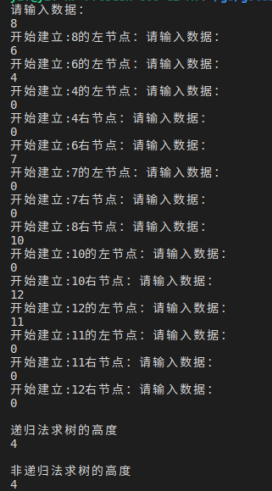完整代码如下：

#include<iostream>
#include<queue>
using namespace std;

// 树(节点)定义
struct TreeNode
{
int		    data; // 值
TreeNode*	left; // 左节点
TreeNode*	right;// 右节点
};

// 按照前序建立二叉树，这里我们建立下面的树
//			8
//		6		10
//	  4	  7			12
//                11
// 所以输入顺序是：8 6 4 0 0 7 0 0 10 0 12 11 0 0 0
void createTree(TreeNode*& t)
{
cout<<"请输入数据："<<endl;
int val = 0;
cin>>val;

// 0表结束
if (0 == val)
{
t = NULL;
}
else
{
t = new TreeNode();
t->data = val;
cout<<"开始建立:"<<val<<"的左节点：";
createTree(t->left);
cout<<"开始建立:"<<val<<"右节点：";
createTree(t->right);
}
}

// Height_Recursive 递归法求树的高度
int Height_Recursive(TreeNode* pTree) {
if (pTree == NULL)
{
return 0;
}
// 分别求出左子树,右子树的高度,取最大值+1
int leftHeight = Height_Recursive(pTree->left);
int rightHeight = Height_Recursive(pTree->right);
return (leftHeight > rightHeight ? leftHeight: rightHeight)+1;
}

// Height_No_Recursive 非递归法求树的高度
// 思路:借鉴层序遍历的思想,一层层的遍历,层数即高度
int Height_No_Recursive(TreeNode* pTree) {
if (pTree == NULL)
{
return 0;
}
queue<TreeNode*> que;
que.push(pTree);
int height = 0;
TreeNode* p = NULL;
while(!que.empty())
{
height++;
// 该层的节点个数,压入所有节点的孩子节点
int num = que.size();
for (int i=0;i<num;i++)
{
p = que.front();
que.pop();

if (p->left != NULL)
{
que.push(p->left);
}
if (p->right != NULL)
{
que.push(p->right);
}
}
}
return height;
}

int main()
{
TreeNode* pTree = NULL;
createTree(pTree);

cout<<"\n递归法求树的高度"<<endl;
cout<<Height_Recursive(pTree)<<endl;

cout<<"\n非递归法求树的高度"<<endl;
cout<<Height_No_Recursive(pTree)<<endl;

return 0;
}


展开全文树的高度 二叉树高度
• 递归法思路： 建立一个数组,count表第1层节点总数,即宽度,count表第二层节点总数,依次类推 用先序遍历二叉树,每深入一层就把该层的节点个数加1,最大节点数即树的宽度 代码如下： // Width_No_Recursive 非...
递归法思路：

建立一个数组,count表第1层节点总数,即宽度,count表第二层节点总数,依次类推
用先序遍历二叉树,每深入一层就把该层的节点个数加1,最大节点数即树的宽度

代码如下：

// Width_No_Recursive 非递归法求树的宽度
// 思路:借鉴层序遍历的思想,一层层的遍历,所有层中最大宽度,即树的宽度
int Width_No_Recursive(TreeNode* pTree) {
if (pTree == NULL)
{
return 0;
}

queue<TreeNode*> que;
que.push(pTree);

int max_weight = 1;
TreeNode* p = NULL;
while(!que.empty())
{
// 该层的节点个数,即该层的宽度
// 弹出该层的节点后,再压入所有节点的孩子节点,即下一层的节点
int num = que.size();
if (num > max_weight)
{
max_weight = num;
}
for (int i=0;i<num;i++)
{
p = que.front();
que.pop();

if (p->left != NULL)
{
que.push(p->left);
}
if (p->right != NULL)
{
que.push(p->right);
}
}
}
return max_weight;
}

非递归法思路：

借鉴层序遍历的思想,一层层的遍历,所有层中最大宽度,即树的宽度

代码如下：

// 统计第level层的节点树
void width(TreeNode* pTree, int* count, int level, int& max)
{
if (pTree == NULL)
{
return;
}
// 该层次节点数加1
count[level]++;
// max一直保存最大宽度
if (count[level] > max)
{
max = count[level];
}
// 往下层递归
width(pTree->left,count,level+1,max);
width(pTree->right,count,level+1,max);
}

// Width_Recursive 递归法求树的宽度
// 思路:建立一个数组,count表第1层节点总数,即宽度,count表第二层节点总数,依次类推
// 用先序遍历二叉树,每深入一层就把该层的节点个数加1,最大节点数即树的宽度
int Width_Recursive(TreeNode* pTree)
{
if (pTree == NULL)
{
return 0;
}
int count = {0};   // 全局数组
int max = 0;            // 宽度

//递归求树的最大宽度
width(pTree,count,1,max);
return max;
}

实验结果如下图：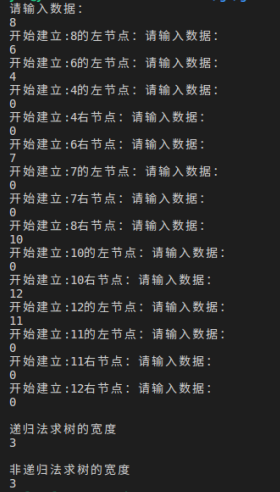完整代码如下：

#include<iostream>
#include<queue>
using namespace std;

// 树(节点)定义
struct TreeNode
{
int		    data; // 值
TreeNode*	left; // 左节点
TreeNode*	right;// 右节点
};

// 按照前序建立二叉树，这里我们建立下面的树
//			8
//		6		10
//	  4	  7			12
//                11
// 所以输入顺序是：8 6 4 0 0 7 0 0 10 0 12 11 0 0 0
void createTree(TreeNode*& t)
{
cout<<"请输入数据："<<endl;
int val = 0;
cin>>val;

// 0表结束
if (0 == val)
{
t = NULL;
}
else
{
t = new TreeNode();
t->data = val;
cout<<"开始建立:"<<val<<"的左节点：";
createTree(t->left);
cout<<"开始建立:"<<val<<"右节点：";
createTree(t->right);
}
}

// 统计第level层的节点树
void width(TreeNode* pTree, int* count, int level, int& max)
{
if (pTree == NULL)
{
return;
}
// 该层次节点数加1
count[level]++;
// max一直保存最大宽度
if (count[level] > max)
{
max = count[level];
}
// 往下层递归
width(pTree->left,count,level+1,max);
width(pTree->right,count,level+1,max);
}

// Width_Recursive 递归法求树的宽度
// 思路:建立一个数组,count表第1层节点总数,即宽度,count表第二层节点总数,依次类推
// 用先序遍历二叉树,每深入一层就把该层的节点个数加1,最大节点数即树的宽度
int Width_Recursive(TreeNode* pTree)
{
if (pTree == NULL)
{
return 0;
}
int count = {0};   // 全局数组
int max = 0;            // 宽度

//递归求树的最大宽度
width(pTree,count,1,max);
return max;
}

// Width_No_Recursive 非递归法求树的宽度
// 思路:借鉴层序遍历的思想,一层层的遍历,所有层中最大宽度,即树的宽度
int Width_No_Recursive(TreeNode* pTree) {
if (pTree == NULL)
{
return 0;
}

queue<TreeNode*> que;
que.push(pTree);

int max_weight = 1;
TreeNode* p = NULL;
while(!que.empty())
{
// 该层的节点个数,即该层的宽度
// 弹出该层的节点后,再压入所有节点的孩子节点,即下一层的节点
int num = que.size();
if (num > max_weight)
{
max_weight = num;
}
for (int i=0;i<num;i++)
{
p = que.front();
que.pop();

if (p->left != NULL)
{
que.push(p->left);
}
if (p->right != NULL)
{
que.push(p->right);
}
}
}
return max_weight;
}

int main()
{
TreeNode* pTree = NULL;
createTree(pTree);

cout<<"\n递归法求树的宽度"<<endl;
cout<<Width_Recursive(pTree)<<endl;

cout<<"\n非递归法求树的宽度"<<endl;
cout<<Width_No_Recursive(pTree)<<endl;

return 0;
}


展开全文二叉树宽度
•tree null struct
• 递归法遍历二叉树 遍历二叉树是指按某条搜索路径巡访树中的每个结点，使得每个结点均被访问一次，而且仅被访问一次 访问的含义很广，可以是对结点做各种处理，包括输出结点的信息，对结点进行运算和修改等 先序...数据结构 算法
• 递归法和非递归法实现n的阶乘 #include &lt;stdio.h&gt; int fac(int n) //递归 { if(n&lt;0) { printf("n&lt;0,data error!\n"); } else if(n==0 || n==1) return 1; else ...C语言
•   今天想来分享一下用C语言求斐波那契数的方法。这里引用一下百度中对斐波那契数的定义。   斐波那契数列指的是这样一个数列 1, 1, 2, 3, 5, 8, 13, 21, 34, 55, 89, 144, 233，377，610，987，1597，2584，...
• 文档：贪心算法引申的非常规货币的凑钱问题，挂载的源码下载链接，通过贪心算法衍生至递归法解决硬币凑钱问题。 博客地址:https://blog.csdn.net/sinat_24470525/article/details/84635451
• 1.递归法 #include #include int fib(int n) {if(n) return 1; else return fib(n-1)+fib(n-2); } int main() {int n=0; printf("请输入n"); scanf("%d\n",&n); system("pause"); return 0; } 2.非递归法 #include...
• 递归法取排列组合易语言源码例程.rar 递归法取排列组合易语言源码例程.rar 递归法取排列组合易语言源码例程.rar 递归法取排列组合易语言源码例程.rar 递归法取排列组合易语言源码例程.rar 递归法取排列组合...
• public class 递归法求和  { /** * @param 递归法求和 */ public static void main(String[] args)  { int n = 100; System.out.print("1+~+"+n+"="); System.out.println(sum(n)); } static int sum(int...java
• 直接就是有一个很清晰的逻辑，第一步干什么，第二部g
• 提交内容只有 该需要实现的函数,不需要main难度系数1 递归法求两个数的最大公约数 (5分) 递归法求两个数的最大公约数。其中 m 和 n 都是用户传入的参数。函数用递归法求m 和 n的最大公约数</p>c语言
• 递归法 unsigned long long Fibonacci(unsigned long long n) { int n1, n2, temp, i; if (n > 2) for (n1 = 1, n2 = 1, i = 3; i ; i++) { temp = n1 + n2; n1 = n2; n2 = temp; } else n2 =...c 斐波那契数列
• 主要介绍了java递归法求字符串逆序,涉及java递归调用的相关操作技巧,需要的朋友可以参考下
• 1知识点：分治递归法求最大子段和顺序表应用7：最大子段和之分治递归法——SDUT题目链接 Time Limit: 10MS Memory Limit: 400KBProblem Description 给定n(1)个整数（可能为负数）组成的序列a,a,a,…,a...最大子段和
• title:C语言学习笔记：普通递归法与尾递归法求n的阶乘的比较 1.普通递归法 #include <stdio.h> unsigned long Fact(unsigned int i); int main() { unsigned int n; printf("please input n:\n"); scanf("%...算法 c语言
• 二叉树的前序遍历：根->左->右1、递归方法：思路：我们知道递归就是将一个大问题不断分成子...例如下面这个二叉树递归过程：转化成代码代码：void PrevOrder() //1、递归法 { Node* root = _Root; _PrevOrder(roo编程题 数据结构...